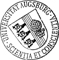## Nonequilibrium effects in the Casimir force between two similar metallic plates kept at different temperatures

• We study the Casimir pressure between two similar plates of finite thickness kept at different temperatures in the case when the dielectric permittivity of the plates depends on temperature. It is suggested to consider the dielectric permittivity at two different temperatures as the permittivities of two dissimilar bodies, thus, allowing to apply the theory of Casimir forces out of thermal equilibrium developed earlier in the literature. Following this approach, we show that, in addition to the equilibrium contribution to the nonequilibrium Casimir pressure, a proper nonequilibrium contribution arises for temperature-dependent dielectric permittivities. Furthermore, the equilibrium contribution in this case does not equal the mean of the equilibrium Casimir pressures at the temperatures of the plates. As an application, the total nonequilibrium Casimir pressure between two gold plates and between two titanium plates is calculated as a function of the plate thickness and theirWe study the Casimir pressure between two similar plates of finite thickness kept at different temperatures in the case when the dielectric permittivity of the plates depends on temperature. It is suggested to consider the dielectric permittivity at two different temperatures as the permittivities of two dissimilar bodies, thus, allowing to apply the theory of Casimir forces out of thermal equilibrium developed earlier in the literature. Following this approach, we show that, in addition to the equilibrium contribution to the nonequilibrium Casimir pressure, a proper nonequilibrium contribution arises for temperature-dependent dielectric permittivities. Furthermore, the equilibrium contribution in this case does not equal the mean of the equilibrium Casimir pressures at the temperatures of the plates. As an application, the total nonequilibrium Casimir pressure between two gold plates and between two titanium plates is calculated as a function of the plate thickness and their separation using the Drude and the plasma models. For plate separations ranging from 0.5 to 2μm, the relative difference between the theoretical predictions for these two models reaches 39%. The proper nonequilibrium term may be as large as 4% of the magnitude of the total nonequilibrium pressure.Author: Gert-Ludwig IngoldORCiDGND, G. L. KlimchitskayaORCiD, V. M. MostepanenkoORCiD urn:nbn:de:bvb:384-opus4-721592 https://opus.bibliothek.uni-augsburg.de/opus4/72159 Physical Review A APS Article English 2020 Universität Augsburg 2020/03/12 101 3 032506 https://doi.org/10.1103/PhysRevA.101.032506 Mathematisch-Naturwissenschaftlich-Technische Fakultät Mathematisch-Naturwissenschaftlich-Technische Fakultät / Institut für Physik Mathematisch-Naturwissenschaftlich-Technische Fakultät / Institut für Physik / Lehrstuhl für Theoretische Physik I 5 Naturwissenschaften und Mathematik / 53 Physik / 530 PhysikDeutsches Urheberrecht